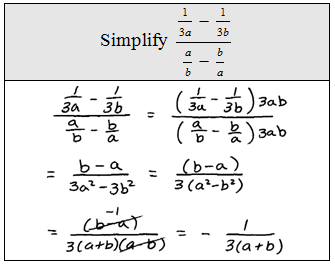## Pages

### Complex Rational Expressions

It turns out that we have all the tools necessary to simplify complex algebraic fractions.

Rational Expressions and Equations Playlist on YouTube

The numerator and denominator of these rational expressions contain fractions and look very intimidating.  We will outline two methods for simplifying them.

Method 1: Obtain a common denominator for the numerator and denominator, multiply by the reciprocal of the denominator, then factor and cancel if possible.Method 2: Multiply the numerator and denominator of the complex fraction by the LCD of all the simple fractions then factor and cancel if possible.To illustrate what happened after we multiplied by the LCD we could add an extra step.For the following solved problems, both methods are used. Choose whichever method feels most comfortable for you.

Simplify using method 1. Simplify using method 2.